# Mean

## Introduction

“What is the age of the students in Grade 8?”

The actual data would be somewhat like the one given below.

 Age of the student Number of students $$13$$ $$10$$ $$14$$ $$16$$ $$15$$ $$4$$

We can conclude that the average age of the students in Grade 8 is $$13.$$ In day-to-day life, it would be easy to use this piece of information rather than the one tabulated above. The ease of using the information gives birth to the measures of central tendency. The three most common measures of central tendency are mean, median and mode.

Know more about the measures of central tendency.
Learn about the Median and the Mode.

## Mean

This is the most common central tendency we know about and use. It is also known as average. It is simply the sum of all the items in a list or collection, divided by the number of items. The mean is represented as $$x$$-bar, $$x̄.$$

### Case 1:

Let there be $$n$$ number of items in a list.

{$${x_1, x_2, x_3, … , x_n }$$}

Mean can be calculated using the formula given below.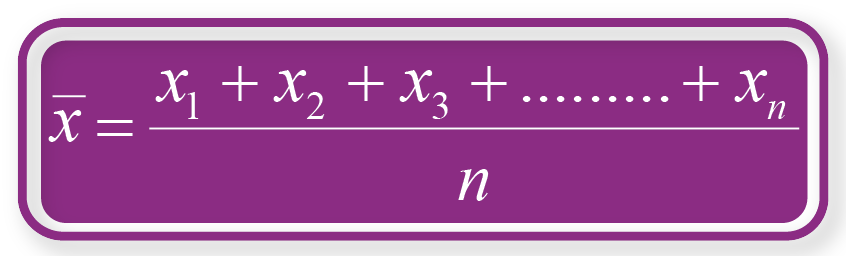OR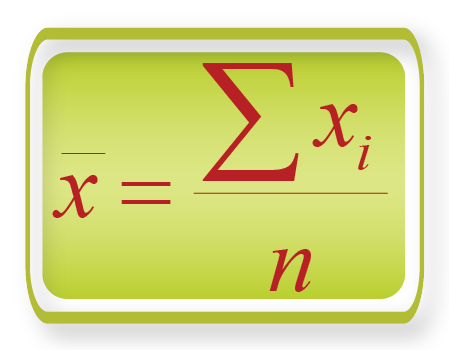### Case 2:

Let there be $$n$$ number of items in a list.

{$${x_1, x_2, x_3, … , x_n }$$}

Let the frequency of each item be

{$${f_1, f_2, f_3, … , f_n }$$} respectively.

Mean can be calculated using the formula given below.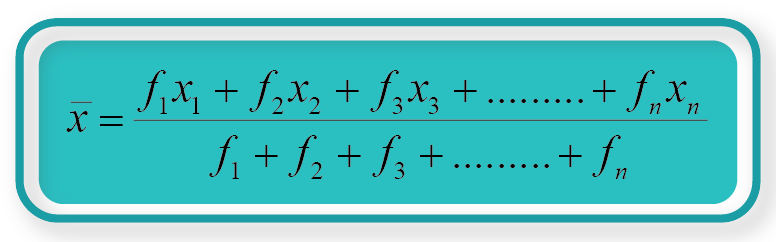OR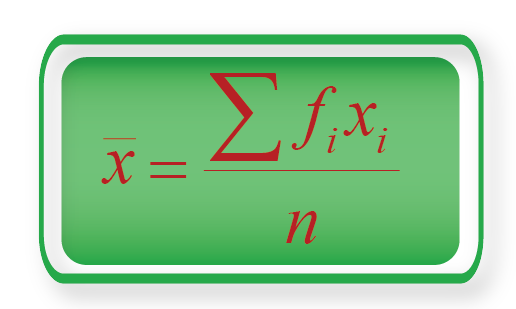### Case 3:

When the items in a list are written in the form of a range, for example $$10\text{-}20,$$ we need to first calculate the class mark.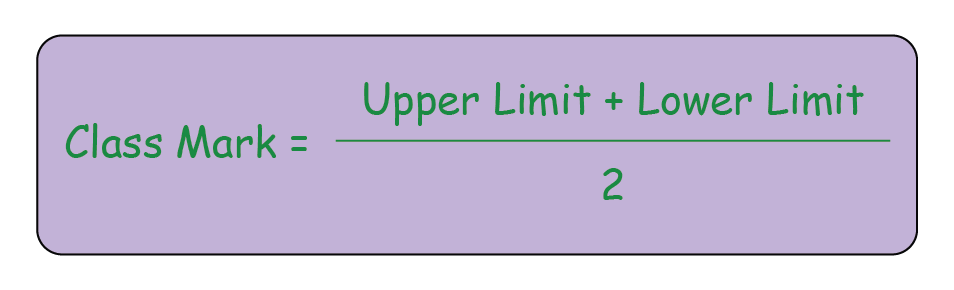Then, the mean can be calculated using the formula given below, where xi will be the class mark for each item.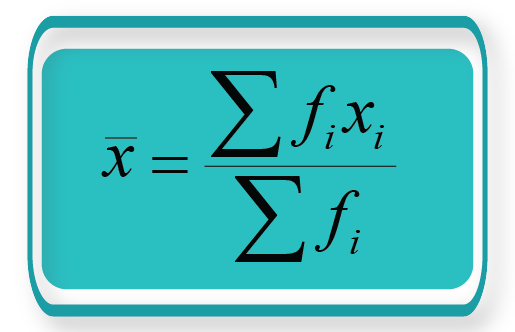## Solved Examples

### Example 1

The following lists the ages of a group of $$10$$ people.

$$\text{A} =$$ {$$45,\,39,\,53,\,45,\,43,\,48,\,50,\,40,\,40,\,45$$}

Calculate the average/mean age of the group.

### Solution:

The total number of people in the group $$=10$$
$$n=10$$

$$x_1=45;\;x_2=39;\;x_3=53;\;…;\;x_n=45$$

We will use the formula given below.\begin{align}\text{The average age of the group}&= \frac{(45 + 39 + 53 + 45 + 43 + 48 + 50 + 40 + 40 + 45)} {10}\\&= \frac{448} {10}\\&= 44.8 \end{align}

\begin{align} \boxed{ \;\;\; \text{Average age of the group}=44.8\;\;\;} \end{align}

### Example 2

There are $$30$$ students in Grade 8. The marks obtained by the students in mathematics are tabulated below. Calculate the mean marks.

 Marks Obtained Number of students $$100$$ $$2$$ $$95$$ $$7$$ $$88$$ $$10$$ $$76$$ $$6$$ $$69$$ $$5$$

### Solution:

The total number of students in Grade 8 $$= 30$$

\begin{align} &x_1=100; \;\;x_2=95; \;\;x_3=88; \;\;x_4=76; \;\;x_5=69\\\\ &f_1=2; \;\;f_2=7; \;\;f_3=10; \;\;f_4=6; \;\;f_5=5\\ \end{align}

\begin{align} &x_1f_1= 100 * 2= 200\\ &x_2f_2 = 95 * 7 \;\, = 665\\ &x_3f_3 = 88 * 10 = 880\\ &x_1f_1 = 76 * 6 \;\,= 456\\ &x_1f_1 = 69 * 5 \;\,= 345 \end{align}

\begin{align} f_1x_1 + f_2x_2 + f_3x_3 + f_4x_4 + f_5x_5 & = 200 + 665 + 880 + 456 + 345 \\ &= 2,546 \\ f_1 + f_2 + f_3 + f_4 + f_5 & = 2 + 7 + 10 + 6 + 5\\ &= 30 \end{align}

We will use the formula given below.\begin{align} \text{Mean marks}&= \frac{2546} {30} \\&= 84.87 \end{align}

\begin{align} \boxed{ \;\;\; \text{Mean marks}= 84.87\;\;\;} \end{align}

### Example 3

There are $$100$$ members in a basketball club. The different age groups of the members and the number of members in each age group are tabulated below. Calculate the mean age of the club members.

 Age Group Number of members $$10\text{-}20$$ $$17$$ $$20\text{-}30$$ $$22$$ $$30\text{-}40$$ $$20$$ $$40\text{-}50$$ $$21$$ $$50\text{-}60$$ $$20$$

### Solution:

In this case we first need to calculate the Class Mark for each age group.

We will use the formula given below and calculate the Class Mark for each age group.Age Group Class Mark Number of members $$10\text{-}20$$ $$15$$ $$17$$ $$20\text{-}30$$ $$25$$ $$22$$ $$30\text{-}40$$ $$35$$ $$20$$ $$40\text{-}50$$ $$45$$ $$21$$ $$50\text{-}60$$ $$55$$ $$20$$

Now,

\begin{align} &x_1=15;\;\;x_2=25;\;\;x_3=35;\;\;x_4=45;\;\;x_5=55 \\ &f_1=17;\;\,f_2=22;\;\;f_3=20;\;\;f_4=21;\;\;f_5=20 \end{align}

\begin{align} &x_1f_1=15*17=255\\ & x_2f_2= 25*22= 550\\ & x_3f_3=35*20=700\\ & x_1f_1=45*21=945\\ & x_1f_1=55*20=1100 \end{align}

\begin{align} f_1x_1 + f_2x_2 + f_3x_3 + f_4x_4 + f_5x_5 &= 255 + 550 + 700 + 945 + 1100 \\&= 3550 \\ f_1 + f_2 + f_3 + f_4 + f_5&= 17 + 22 + 20 + 21 + 20\\ &= 100 \end{align}

We will use the formula given below.\begin{align} \text{The mean age}&= \frac{3550} {100}\\&= 35.5 \end{align}

\begin{align} \boxed{\;\;\; \text{The mean age of the members}=35.5 \;\;\;} \end{align}

## Practice Questions

### Question 1

The following lists the height of a group of $$10$$ people in centimetres.
$$A =$${$$145, 139, 153, 145, 143, 148, 150, 140, 140, 145$$}

Calculate the average / mean height of the group.

Hint: Use the formula### Question 2

There are $$40$$ students in Grade 10. The marks obtained by the students in English are tabulated below. Calculate the mean marks.

 Marks Obtained Number of students $$100\text{-}90$$ $$5$$ $$90\text{-}80$$ $$10$$ $$80\text{-}70$$ $$15$$ $$70\text{-}60$$ $$6$$ $$60\text{-}50$$ $$4$$

Hint: Use the formulaeand### Question 3

There are $$20$$ families residing in an apartment complex. The table given below shows the number of cars owned by the families. Calculate the mean number of cars owned by each family.

 Number of cars Number of families $$4$$ $$3$$ $$3$$ $$3$$ $$2$$ $$5$$ $$1$$ $$9$$

Hint: Use the formulaeMore Important Topics
Numbers
Algebra
Geometry
Measurement
Money
Data
Trigonometry
Calculus
More Important Topics
Numbers
Algebra
Geometry
Measurement
Money
Data
Trigonometry
Calculus
Learn from the best math teachers and top your exams

• Live one on one classroom and doubt clearing
• Practice worksheets in and after class for conceptual clarity
• Personalized curriculum to keep up with school